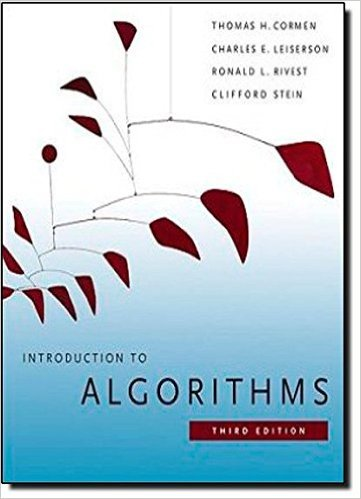×
Get Full Access to Introduction To Algorithms - 3 Edition - Chapter 15 - Problem 15-12
Get Full Access to Introduction To Algorithms - 3 Edition - Chapter 15 - Problem 15-12

×

# Signing free-agent baseball players Suppose that you areISBN: 9780262033848 130

## Solution for problem 15-12 Chapter 15

Introduction to Algorithms | 3rd Edition

• Textbook Solutions
• 2901 Step-by-step solutions solved by professors and subject experts
• Get 24/7 help from StudySoup virtual teaching assistantsIntroduction to Algorithms | 3rd Edition

4 5 1 366 Reviews
12
3
Problem 15-12

Signing free-agent baseball players Suppose that you are the general manager for a major-league baseball team. During the off-season, you need to sign some free-agent players for your team. The team owner has given you a budget of \$X to spend on free agents. You are allowed to spend less than \$X altogether, but the owner will fire you if you spend any more than \$X. You are considering N different positions, and for each position, P free-agent players who play that position are available.8 Because you do not want to overload your roster with too many players at any position, for each position you may sign at most one free agent who plays that position. (If you do not sign any players at a particular position, then you plan to stick with the players you already have at that position.) To determine how valuable a player is going to be, you decide to use a sabermetric statistic9 known as VORP, or value over replacement player. A player with a higher VORP is more valuable than a player with a lower VORP. A player with a higher VORP is not necessarily more expensive to sign than a player with a lower VORP, because factors other than a players value determine how much it costs to sign him. For each available free-agent player, you have three pieces of information: the players position, the amount of money it will cost to sign the player, and the players VORP. Devise an algorithm that maximizes the total VORP of the players you sign while spending no more than \$X altogether. You may assume that each player signs for a multiple of \$100,000. Your algorithm should output the total VORP of the players you sign, the total amount of money you spend, and a list of which players you sign. Analyze the running time and space requirement of your algorithm.

Step-by-Step Solution:
Step 1 of 3

Math246 Week 8: Complex Roots Now we will look at differential equations with complex roots. This involves equations of the form ay +by +cy=0 . 2 w=κ±γi The roots of the quadratic equation aw +bw+c=0 are (κ+γi)t (−γi)t The general solution is thus y (t=e and y( )=e But this is pretty ugly so we will use Euler’s formula to make it into something nicer. e =cos (θ +isin (θ) −iθ e =cos (−θ +isin (−θ =cos (θ)−isin(θ) So our two general solutions become y(t)=e (cos (γt +isin (γt ) κt y(t)=e (cos (γt −isin (γt)) Now if we add the two equations together and divide by two, we get e cos (γt)

Step 2 of 3

Step 3 of 3